Function Repository Resource:

# OrderedSchurDecomposition

Compute the ordered Schur decomposition of a matrix

Contributed by: Jan Mangaldan
 ResourceFunction["OrderedSchurDecomposition"][m] yields the ordered Schur decomposition for a numerical matrix m, given as a list {q,t} where q is an orthonormal matrix and t is a block upper‐triangular matrix. ResourceFunction["OrderedSchurDecomposition"][{m,a}] gives the generalized Schur decomposition of the matrix pencil {m,a}, where m and a are numerical matrices.

## Details and Options

ResourceFunction["OrderedSchurDecomposition"] is similar to the built-in SchurDecomposition, except that the diagonal blocks in t are sorted according to a specified order.
The matrix m must be square.
The original matrix m is equal to q.t.ConjugateTranspose[q].
In ResourceFunction["OrderedSchurDecomposition"][{m,a}], the matrices m and a must be square, and have the same size.
ResourceFunction["OrderedSchurDecomposition"][{m,a}] yields a list of matrices {q,s,p,t} where q and p are orthonormal matrices, and s and t are upper‐triangular matrices, such that m is given by q.s.ConjugateTranspose[p], and a is given by q.t.ConjugateTranspose[p].
ResourceFunction["OrderedSchurDecomposition"] has the following options and settings:
 "Criteria" "Magnitude" criterion used for ordering diagonal elements in the triangular factor "Order" "Decreasing" whether to sort in increasing or decreasing order Pivoting False whether to perform pivoting RealBlockDiagonalForm True whether to use real blocks instead of complex values for real matrices
The option "Criteria" can be set to any of the following:
 "Magnitude" sort diagonal elements by magnitude "RealPart" sort diagonal elements by their real parts "AbsRealPart" sort diagonal elements by the absolute values of their real parts "AbsImaginaryPart" sort diagonal elements by the absolute values of their imaginary parts
The option "Order" can be set to any of the following:
 "Decreasing" sort in decreasing order "Increasing" sort in increasing order
With the setting PivotingTrue, ResourceFunction["OrderedSchurDecomposition"] yields a list {q,t,d} where d is a permuted diagonal matrix such that m.d is equal to d.q.t.ConjugateTranspose[q].
For real-valued matrices m, setting the option allows complex values on the diagonal of the t matrix.

## Examples

### Basic Examples (2)

The ordered Schur decomposition of a real matrix:

 In:=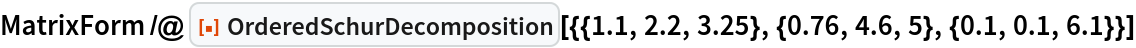Out=Compare with the result of SchurDecomposition:

 In:=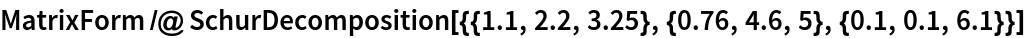Out=### Scope (3)

A 4×4 matrix with real entries:

 In:=Compute the ordered Schur decomposition with machine precision, with the diagonal elements sorted in decreasing order of magnitude:

 In:=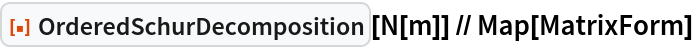Out=Compute the ordered Schur decomposition with 24-digit arbitrary precision:

 In:=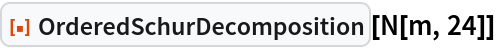Out=Sort the diagonal elements by their real parts, in increasing order:

 In:=Out=A 3×3 matrix with complex entries:

 In:=Ordered Schur decomposition of a complex matrix, with the diagonal elements sorted in decreasing order of magnitude:

 In:=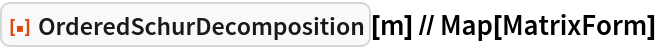Out=Sort the diagonal elements by the magnitude of their imaginary parts, in increasing order:

 In:=Out=Compute the ordered generalized Schur decomposition for a matrix pencil:

 In:=Out=Use a different ordering:

 In:=Out=### Options (13)

#### Criteria (4)

m is a matrix with complex eigenvalues:

 In:=Out=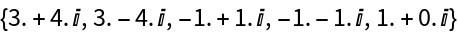Sort the diagonal elements by their magnitude in decreasing order:

 In:=In:=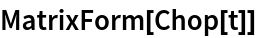Out=Sort the diagonal elements by their real parts in decreasing order:

 In:=In:=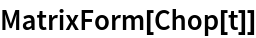Out=Sort the diagonal elements by the magnitude of their imaginary parts:

 In:=In:=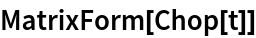Out=#### Order (3)

m is a matrix with positive eigenvalues:

 In:=Out=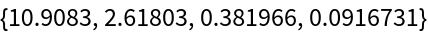Sort the diagonal elements in decreasing order:

 In:=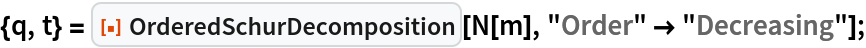In:=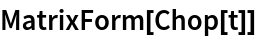Out=Sort the diagonal elements in increasing order:

 In:=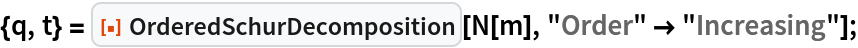In:=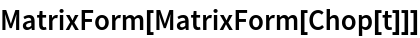Out=#### Pivoting (3)

m is a 3×3 matrix:

 In:=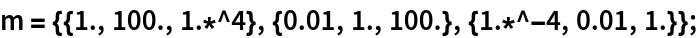With PivotingTrue, an extra matrix that represents the scaling and permutation is returned:

 In:=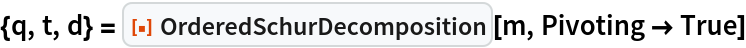Out=In:=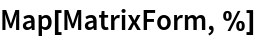Out=Verify that m.d is equal to d.q.t.ConjugateTranspose[q]:

 In:=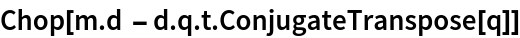Out=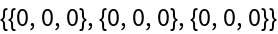#### RealBlockDiagonalForm (3)

m is a matrix with two real and two complex eigenvalues:

 In:=Out=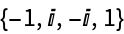With , the result is complex upper triangular:

 In:=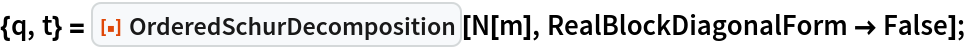In:=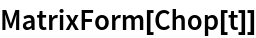Out=With , there are real 2×2 blocks along the diagonal:

 In:=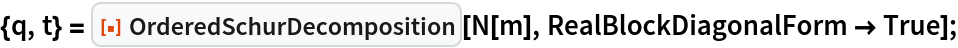In:=Out=### Properties and Relations (2)

m is a matrix with two real and two complex eigenvalues:

 In:=Out=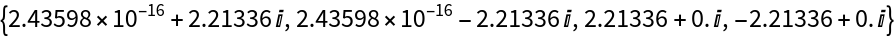Find the ordered Schur decomposition of m:

 In:=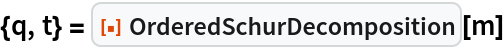Out=The real eigenvalues appear on the diagonal of t, the complex as a 2×2 block:

 In:=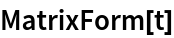Out=Verify that m is equal to q.t.ConjugateTranspose[q]:

 In:=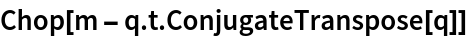Out=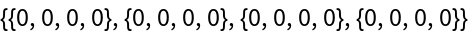A matrix pencil:

 In:=Find the ordered generalized Schur decomposition:

 In:=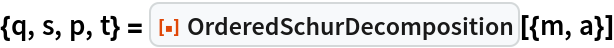Out=Verify that m is given by q.s.ConjugateTranspose[p]:

 In:=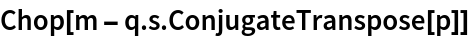Out=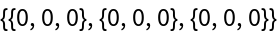Verify that a is given by q.t.ConjugateTranspose[p]:

 In:=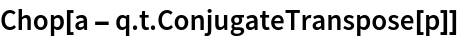Out=## Version History

• 1.0.0 – 07 February 2022